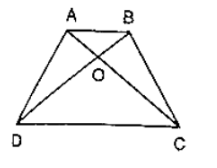# In figure, ABCD is a trapezium in which AB ∥ DC. Prove that ar(ΔAOD) = ar(ΔBOC)

Question:

In figure, ABCD is a trapezium in which AB ∥ DC. Prove that ar(ΔAOD) = ar(ΔBOC)Solution:

Given:  ABCD is a trapezium in which AB ∥ DC

To prove: ar(ΔAOD) = ar(ΔBOC)

Proof: Since, ΔADC and ΔBDC are on the same base DC and between same parallels AB and DC